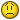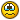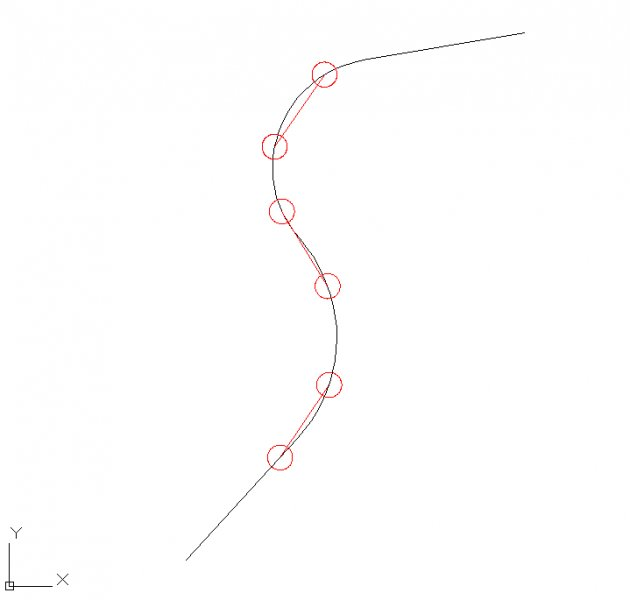# 2 points on 1 path## Recommended PostsHello

I tried several ways to let 2 points (cirlces, see image below) following a drawing path (polyline). I tried it with arraypath etc.

It doesn't work and I don't find any solution for this problem. Can somebody help me? I work with Autocad 2013.

The two circles must follow the polylinepath always with the same distance between the circles. It's like a train or tram movement. The user must be able to enter a certain distance between the objects that has to be copied and the user must also be able to chose how many times it has to be copied.

Please, does anyone know a solution?Thx in advance.##### Share on other sitesmeasure command

##### Share on other sitesmeasure command

I tried that too but it doesn't work. Always one circle doesn't follow the path.##### Share on other sitesDo, but insert blocks or AutoCAD dots (points). And do very well.

Attached drawing in which you try

##### Share on other sitesmeasure command

I would have thought that measure would give equal lengths along the curve, but not what the OP requires, which is an equal chord length.

I would probably use a suitable block and rotate it for required alignment.

##### Share on other sitesThere is a way you place a block on the pline and the 1st insertion pt is cen of circle 2nd rotation pt is calculated and block rotated to this angle, the code is in VBA but I would do now in VL the variable crad is the distance between centres to try make a block called "holden"

```Sub draw_vehicle()
Dim CAR As String
Dim retVal As Variant
Dim snapPt As Variant
Dim oCoords As Variant
Dim blpnt1() As Variant
ReDim blpnt1(100)
Dim blpnt2() As Variant
ReDim blpnt2(100)
Dim vertPt(0 To 2) As Double
Dim Pt1(0 To 2) As Double
Dim Pt2(0 To 2) As Double
Dim newPt(0 To 2) As Double
Dim iCnt, w, x, y, z As Integer
Dim cRad, interval, blkangle As Double
On Error GoTo Something_Wrong
If ThisDrawing.ActiveSpace = acModelSpace Then
Set Thisspace = ThisDrawing.ModelSpace
Else: Set Thisspace = ThisDrawing.PaperSpace
End If
For Each Item In ThisDrawing.Blocks
If Item.Name = "holden" Then GoTo continue_on
Next Item
' insert holden block
InsertBlock "p:\Autodesk\vba\holdencar.dwg", 0
continue_on:
w = 1
ThisDrawing.Utility.GetEntity oPoly, snapPt, vbCr & "Select polyline :"
If oPoly.ObjectName = "AcDbPolyline" Then
oCoords = oPoly.Coordinates
Else: MsgBox "This object is not a polyline! Please do again"
Exit Sub
End If
interval = CDbl(InputBox("Enter interval:", , 1#))
If interval < 1 Then
interval = 1
End If
For iCnt = 0 To UBound(oCoords) - 2 Step 2
Pt1(0) = oCoords(iCnt): Pt1(1) = oCoords(iCnt + 1): Pt1(2) = 0#
newPt(0) = Pt1(0)
newPt(1) = Pt1(1)
newPt(2) = 0#
iCnt = iCnt + 2
Pt2(0) = oCoords(iCnt): Pt2(1) = oCoords(iCnt + 1): Pt2(2) = 0#
x = (Pt1(0) - Pt2(0)) / interval
y = (Pt1(1) - Pt2(1)) / interval
'reset back 2 values
iCnt = iCnt - 2
startang = 4.71239
endang = 1.570796
CAR = "HOLDEN"
For z = 1 To interval
vertPt(0) = newPt(0) - x
vertPt(1) = newPt(1) - y
vertPt(2) = 0#
'blpnt1(w) = vertPt
retval2 = arcobj.IntersectWith(oPoly, acExtendOtherEntity)
arcobj.Delete
Set arcobj = Nothing
blkangle = ThisDrawing.Utility.AngleFromXAxis(retval2, vertPt)
'Set blkobj = ThisDrawing.ModelSpace.InsertBlock(vertPt, CAR, 1#, 1#, 1#, blkangle)
Set blkobj = Thisspace.InsertBlock(vertPt, CAR, 1#, 1#, 1#, blkangle)
Set blkobj = Nothing
w = w + 1
newPt(0) = newPt(0) - x
newPt(1) = newPt(1) - y
Next z
Next iCnt
GoTo Exit_out
Something_Wrong:
MsgBox Err.Description
Exit_out:
End Sub
Private Sub InsertBlock1()

'****************************************

'*** Code from VisibleVisual.com ********

'****************************************

InsertBlock "p:\Autodesk\vbaholden.dwg", 0

'Change the 0 to another value (in degrees) to rotate the block'

End Sub

Function InsertBlock(ByVal blockpath As String, ByVal rotation As Double)

Dim insertionPnt As Variant

Dim prompt1 As String

'set rotation Angle

rotateAngle = rotation

'rotateAngle = rotation * 3.141592 / 180#

'Prompt is used to show instructions in the command bar

prompt1 = vbCrLf & "Enter block insert point: "

'ThisDrawing.ActiveSpace = acModelSpace

insertionPnt = ThisDrawing.Utility.GetPoint(, prompt1)

Set blockRefObj = ThisDrawing.ModelSpace.InsertBlock(insertionPnt, blockpath, 1#, 1#, 1#, rotateAngle)

'Change Modelspace into Paperspace to insert the block into Paperspace

End Function
```

##### Share on other sitesDo, but insert blocks or AutoCAD dots (points). And do very well.

Attached drawing in which you try

Hi, below the autocadfile2pointspath.dwg

##### Share on other sitesI would have thought that measure would give equal lengths along the curve, but not what the OP requires, which is an equal chord length.

I would probably use a suitable block and rotate it for required alignment.

That's what I'm doing now. But sometimes it's more than one block and it takes a lot of time and sometimes you snap to the wrong line.

Therefor, with a lisproutine (if it's possible) you don't make a mistake##### Share on other sitesThere is a way you place a block on the pline and the 1st insertion pt is cen of circle 2nd rotation pt is calculated and block rotated to this angle, the code is in VBA but I would do now in VL the variable crad is the distance between centres to try make a block called "holden"

```Sub draw_vehicle()
Dim CAR As String
Dim retVal As Variant
Dim snapPt As Variant
Dim oCoords As Variant
Dim blpnt1() As Variant
ReDim blpnt1(100)
Dim blpnt2() As Variant
ReDim blpnt2(100)
Dim vertPt(0 To 2) As Double
Dim Pt1(0 To 2) As Double
Dim Pt2(0 To 2) As Double
Dim newPt(0 To 2) As Double
Dim iCnt, w, x, y, z As Integer
Dim cRad, interval, blkangle As Double
On Error GoTo Something_Wrong
If ThisDrawing.ActiveSpace = acModelSpace Then
Set Thisspace = ThisDrawing.ModelSpace
Else: Set Thisspace = ThisDrawing.PaperSpace
End If
For Each Item In ThisDrawing.Blocks
If Item.Name = "holden" Then GoTo continue_on
Next Item
' insert holden block
InsertBlock "p:\Autodesk\vba\holdencar.dwg", 0
continue_on:
w = 1
ThisDrawing.Utility.GetEntity oPoly, snapPt, vbCr & "Select polyline :"
If oPoly.ObjectName = "AcDbPolyline" Then
oCoords = oPoly.Coordinates
Else: MsgBox "This object is not a polyline! Please do again"
Exit Sub
End If
interval = CDbl(InputBox("Enter interval:", , 1#))
If interval < 1 Then
interval = 1
End If
For iCnt = 0 To UBound(oCoords) - 2 Step 2
Pt1(0) = oCoords(iCnt): Pt1(1) = oCoords(iCnt + 1): Pt1(2) = 0#
newPt(0) = Pt1(0)
newPt(1) = Pt1(1)
newPt(2) = 0#
iCnt = iCnt + 2
Pt2(0) = oCoords(iCnt): Pt2(1) = oCoords(iCnt + 1): Pt2(2) = 0#
x = (Pt1(0) - Pt2(0)) / interval
y = (Pt1(1) - Pt2(1)) / interval
'reset back 2 values
iCnt = iCnt - 2
startang = 4.71239
endang = 1.570796
CAR = "HOLDEN"
For z = 1 To interval
vertPt(0) = newPt(0) - x
vertPt(1) = newPt(1) - y
vertPt(2) = 0#
'blpnt1(w) = vertPt
retval2 = arcobj.IntersectWith(oPoly, acExtendOtherEntity)
arcobj.Delete
Set arcobj = Nothing
blkangle = ThisDrawing.Utility.AngleFromXAxis(retval2, vertPt)
'Set blkobj = ThisDrawing.ModelSpace.InsertBlock(vertPt, CAR, 1#, 1#, 1#, blkangle)
Set blkobj = Thisspace.InsertBlock(vertPt, CAR, 1#, 1#, 1#, blkangle)
Set blkobj = Nothing
w = w + 1
newPt(0) = newPt(0) - x
newPt(1) = newPt(1) - y
Next z
Next iCnt
GoTo Exit_out
Something_Wrong:
MsgBox Err.Description
Exit_out:
End Sub
Private Sub InsertBlock1()

'****************************************

'*** Code from VisibleVisual.com ********

'****************************************

InsertBlock "p:\Autodesk\vbaholden.dwg", 0

'Change the 0 to another value (in degrees) to rotate the block'

End Sub

Function InsertBlock(ByVal blockpath As String, ByVal rotation As Double)

Dim insertionPnt As Variant

Dim prompt1 As String

'set rotation Angle

rotateAngle = rotation

'rotateAngle = rotation * 3.141592 / 180#

'Prompt is used to show instructions in the command bar

prompt1 = vbCrLf & "Enter block insert point: "

'ThisDrawing.ActiveSpace = acModelSpace

insertionPnt = ThisDrawing.Utility.GetPoint(, prompt1)

Set blockRefObj = ThisDrawing.ModelSpace.InsertBlock(insertionPnt, blockpath, 1#, 1#, 1#, rotateAngle)

'Change Modelspace into Paperspace to insert the block into Paperspace

End Function
```

Thx, but I don't know how to start or how to run a VBA program on my Autocad. Anyway, I hope you will succeed in VL##### Share on other sitesYour problem is more complicated because you have to align block "TEMP" (which does not have the same length in the direction of x and y) from the point of insertion and a calculated point at a distance equal to the length of the block.

Edited by Costinbos77
##### Share on other sitesI am redoing to match "temp"

Any way a quick way insert block make sure insertion point is center of 1 circle then draw a circle 7.0 units rotate the block using Ro R the crossing point is the correct angle.

My version draws lots of blocks its for checking purposes.

Constinboss77 have a look at the code thats what it does rotates the block to suit.

##### Share on other sitesYour problem is more complicated because you have to align block "TEMP" from the point of insertion and a calculated point at a distance equal to the length of the block.

I know and it doesn't work with a normal Autocad commando##### Share on other sitesRevised have a look at this

Type VBAMAN New pick the global1, visual basic editor, double click the global1 top left the code window should open paste code & save to see result use the RUN sub/userform try 1 for interval. Its more about the method of how to do. You will need a new vertices lsp as well to work out how many you display and at what spacing. Time at moment is a problem for a complete solution.

```Sub draw_vehicle()
Dim CAR As String
Dim retVal As Variant
Dim snapPt As Variant
Dim oCoords As Variant
Dim blpnt1() As Variant
ReDim blpnt1(100)
Dim blpnt2() As Variant
Dim vertPt(0 To 2) As Double
Dim Pt1(0 To 2) As Double
Dim Pt2(0 To 2) As Double
Dim newPt(0 To 2) As Double
Dim iCnt, w, x, y, z As Integer
Dim cRad, interval, blkangle As Double
On Error GoTo Something_Wrong
For Each Item In ThisDrawing.Blocks
If Item.Name = "temp" Then GoTo continue_on
Next Item
' exits out of program
GoTo Exit_out
continue_on:
w = 1
ThisDrawing.Utility.GetEntity oPoly, snapPt, vbCr & "Select polyline :"
If oPoly.ObjectName = "AcDbPolyline" Then
oCoords = oPoly.Coordinates
Else: MsgBox "This object is not a polyline!"
Exit Sub
End If
interval = CDbl(InputBox("Enter interval:", , 1#))
If interval < 1 Then
interval = 1
End If
For iCnt = 0 To UBound(oCoords) - 2 Step 2
Pt1(0) = oCoords(iCnt): Pt1(1) = oCoords(iCnt + 1): Pt1(2) = 0#
newPt(0) = Pt1(0)
newPt(1) = Pt1(1)
newPt(2) = 0#
iCnt = iCnt + 2
Pt2(0) = oCoords(iCnt): Pt2(1) = oCoords(iCnt + 1): Pt2(2) = 0#
x = (Pt1(0) - Pt2(0)) / interval
y = (Pt1(1) - Pt2(1)) / interval
'reset back 2 values
iCnt = iCnt - 2
startang = 4.7123889
endang = 1.57079632
CAR = "temp"
For z = 1 To interval
vertPt(0) = newPt(0) - x
vertPt(1) = newPt(1) - y
vertPt(2) = 0#
'blpnt1(w) = vertPt
retval2 = arcobj.IntersectWith(oPoly, acExtendOtherEntity)
arcobj.Delete
Set arcobj = Nothing
blkangle = ThisDrawing.Utility.AngleFromXAxis(retval2, vertPt)
Set blkobj = ThisDrawing.ModelSpace.InsertBlock(vertPt, CAR, 1#, 1#, 1#, blkangle)
Set blkobj = Nothing
w = w + 1
newPt(0) = newPt(0) - x
newPt(1) = newPt(1) - y
Next z
Next iCnt
Something_Wrong:
MsgBox Err.Description
Exit_out:
End Sub
```

## Join the conversation

You can post now and register later. If you have an account, sign in now to post with your account.
Note: Your post will require moderator approval before it will be visible.×   Pasted as rich text.   Restore formatting

Only 75 emoji are allowed.